# An Introduction To Fuzzy Control Systems

v1.0.4 / 01 jun 03 / greg goebel / public domain

* This document is an introduction to fuzzy control systems. It provides an overview of their theory of operation, followed by elementary examples of their use.

##  INTRODUCTION TO FUZZY LOGIC & FUZZY CONTROL

* "Fuzzy logic" has become a common buzzword in machine control. However, the term itself inspires a certain skepticism, sounding equivalent to "half-baked logic" or "bogus logic". Some other nomenclature might have been preferable, but it's too late now, and fuzzy logic is actually very straightforward. Fuzzy logic is a way of interfacing inherently analog processes, that move through a continuous range of values, to a digital computer, that likes to see things as well-defined discrete numeric values.

For example, consider an antilock braking system, directed by a microcontroller chip. The microcontroller has to make decisions based on brake temperature, speed, and other variables in the system.

The variable "temperature" in this system can be divided into a range of "states", such as: "cold", "cool", "moderate", "warm", "hot", "very hot". Defining the bounds of these states is a bit tricky. An arbitrary threshold might be set to divide "warm" from "hot", but this would result in a discontinuous change when the input value passed over that threshold.

The way around this is to make the states "fuzzy", that is, allow them to change gradually from one state to the next. You could define the input temperature states using "membership functions" such as the following: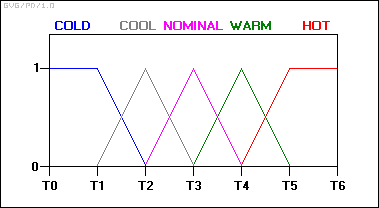With this scheme, the input variable's state no longer jumps abruptly from one state to the next. Instead, as the temperature changes, it loses value in one membership function while gaining value in the next. At any one time, the "truth value" of the brake temperature will almost always be in some degree part of two membership functions: 0.6 nominal and 0.4 warm, or 0.7 nominal and 0.3 cool, and so on.

The input variables in a fuzzy control system are in general mapped into by sets of membership functions similar to this, known as "fuzzy sets". The process of converting a crisp input value to a fuzzy value is called "fuzzification".

A control system may also have various types of switch, or "ON-OFF", inputs along with its analog inputs, and such switch inputs of course will always have a truth value equal to either 1 or 0, but the scheme can deal with them as simplified fuzzy functions that are either one value or another.

Given "mappings" of input variables into membership functions and truth values, the microcontroller then makes decisions for what action to take based on a set of "rules", each of the form:

```   IF brake temperature IS warm AND speed IS not very fast
THEN brake pressure IS slightly decreased.
```
In this example, the two input variables are "brake temperature" and "speed" that have values defined as fuzzy sets. The output variable, "brake pressure", is also defined by a fuzzy set that can have values like "static", "slightly increased", "slightly decreased", and so on.

This rule by itself is very puzzling since it looks like it could be used without bothering with fuzzy logic, but remember the decision is based on a set of rules:

• All the rules that apply are invoked, using the membership functions and truth values obtained from the inputs, to determine the result of the rule.

• This result in turn will be mapped into a membership function and truth value controlling the output variable.

• These results are combined to give a specific ("crisp") answer, the actual brake pressure, a procedure known as "defuzzification".

This combination of fuzzy operations and rule-based "inference" describes a "fuzzy expert system".

Traditional control systems are based on mathematical models in which the the control system is described using one or more differential equations that define the system response to its inputs. Such systems are often implemented as "proportional-integral-derivative (PID)" controllers. They are the products of decades of development and theoretical analysis, and are highly effective.

If PID and other traditional control systems are so well-developed, why bother with fuzzy control? It has some advantages. In many cases, the mathematical model of the control process may not exist, or may be too "expensive" in terms of computer processing power and memory, and a system based on empirical rules may be more effective.

Furthermore, fuzzy logic is well suited to low-cost implementations based on cheap sensors, low-resolution analog-to-digital converters, and 4-bit or 8-bit one-chip microcontroller chips. Such systems can be easily upgraded by adding new rules to improve performance or add new features. In many cases, fuzzy control can be used to improve existing traditional controller systems by adding an extra layer of intelligence to the current control method.

##  FUZZY CONTROL IN DETAIL

* Fuzzy controllers are very simple conceptually. They consist of an input stage, a processing stage, and an output stage. The input stage maps sensor or other inputs, such as switches, thumbwheels, and so on, to the appropriate membership functions and truth values. The processing stage invokes each appropriate rule and generates a result for each, then combines the results of the rules. Finally, the output stage converts the combined result back into a specific control output value.

The most common shape of membership functions is triangular, although trapezoids and bell curves are also used, but the shape is generally less important than the number of curves and their placement. From three to seven curves are generally appropriate to cover the required range of an input value, or the "universe of discourse" in fuzzy jargon.

As discussed earlier, the processing stage is based on a collection of logic rules in the form of IF-THEN statements, where the IF part is called the "antecedent" and the THEN part is called the "consequent". Typical fuzzy control systems have dozens of rules.

Consider a rule for a thermostat:

```   IF (temperature is "cold") THEN (heater is "high")
```
This rule uses the truth value of the "temperature" input, which is some truth value of "cold", to generate a result in the fuzzy set for the "heater" output, which is some value of "high". This result is used with the results of other rules to finally generate the crisp composite output. Obviously, the greater the truth value of "cold", the higher the truth value of "high", though this does not necessarily mean that the output itself will be set to "high", since this is only one rule among many.

In some cases, the membership functions can be modified by "hedges" that are equivalent to adjectives. Common hedges include "about", "near", "close to", "approximately", "very", "slightly", "too", "extremely", and "somewhat". These operations may have precise definitions, though the definitions can vary considerably between different implementations. "Very", for one example, squares membership functions; since the membership values are always less than 1, this narrows the membership function. "Extremely" cubes the values to give greater narrowing, while "somewhat" broadens the function by taking the square root.

In practice, the fuzzy rule sets usually have several antecedents that are combined using fuzzy operators, such as AND, OR, and NOT, though again the definitions tend to vary: AND, in one popular definition, simply uses the minimum weight of all the antecedents, while OR uses the maximum value. There is also a NOT operator that subtracts a membership function from 1 to give the "complementary" function.

There are several different ways to define the result of a rule, but one of the most common and simplest is the "max-min" inference method, in which the output membership function is given the truth value generated by the premise.

Rules can be solved in parallel in hardware, or sequentially in software. The results of all the rules that have fired are "defuzzified" to a crisp value by one of of several methods. There are dozens in theory, each with various advantages and drawbacks.

The "centroid" method is very popular, in which the "center of mass" of the result provides the crisp value. Another approach is the "height" method, which takes the value of the biggest contributor. The centroid method favors the rule with the output of greatest area, while the height method obviously favors the rule with the greatest output value.

The example below demonstrates max-min inferencing and centroid defuzzification for a system with input variables "x", "y", and "z" and an output variable "n". Note that "mu" is standard fuzzy-logic nomenclature for "truth value":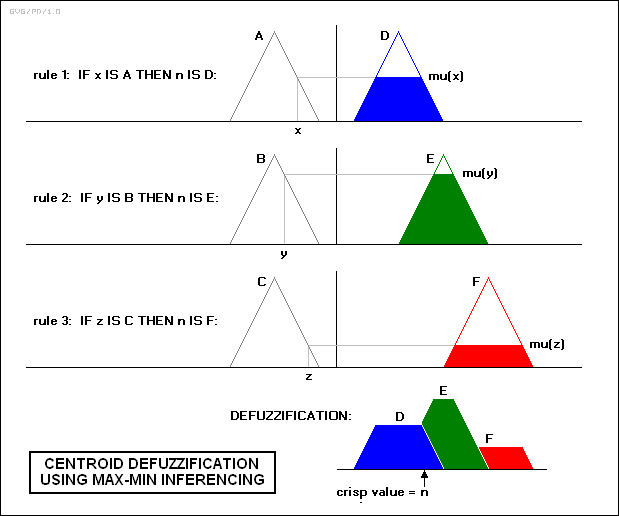Notice how each rule provides a result as a truth value of a particular membership function for the output variable. In centroid defuzzification the values are OR'd, that is, the maximum value is used and values are not added, and the results are then combined using a centroid calculation.

* Fuzzy control system design is based on empirical methods, basically a methodical approach to trial-and-error. The general process is as follows:

• Document the system's operational specifications and inputs and outputs.
• Document the fuzzy sets for the inputs.
• Document the rule set.
• Determine the defuzzification method.
• Run through test suite to validate system, adjust details as required.
• Complete document and release to production.

As a general example, consider the design of a fuzzy controller for a steam turbine. The block diagram of this control system appears as follows: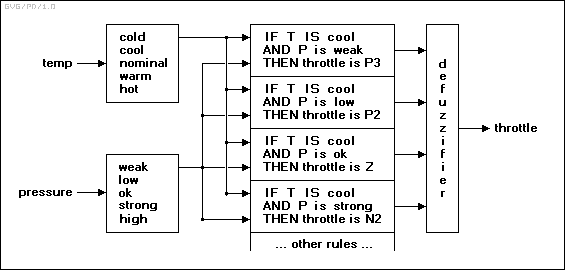There are two input variables, temperature and pressure, and a single output variable, the turbine throttle setting. The turbine's operation can be reversed, so the throttle setting can be positive or negative. The fuzzy set mappings are shown below: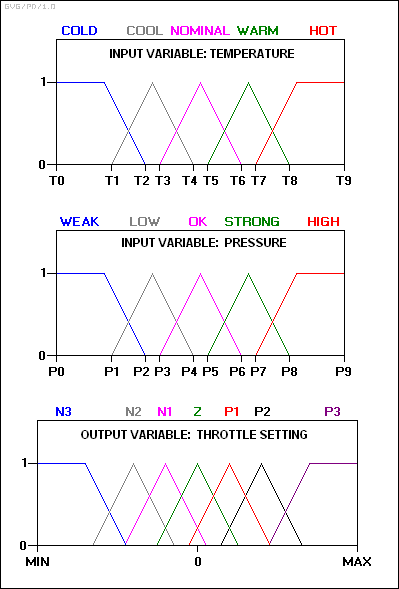The throttle settings are defined as follows:

```   N3:   Large negative.
N2:   Medium negative.
N1:   Small negative.
Z:    Zero.
P1:   Small positive.
P2:   Medium positive.
P3:   Large positive.

```
The rule set includes such rules as:
``` rule 1:  IF temperature IS cool AND pressure IS weak,
THEN throttle is P3.

rule 2:  IF temperature IS cool AND pressure IS low,
THEN throttle is P2.

rule 3:  IF temperature IS cool AND pressure IS ok,
THEN throttle is Z.

rule 4:  IF temperature IS cool AND pressure IS strong,
THEN throttle is N2.

```
In practice, the controller accepts the inputs and maps them into their membership functions and truth values. These mappings are then fed into the rules. If the rule specifies an AND relationship between the mappings of the two input variables, as the examples above do, the minimum of the two is used as the combined truth value; if an OR is specified, the maximum is used. The appropriate output state is selected and assigned a membership value at the truth level of the premise. The truth values are then defuzzified.

For an example, assume the temperature is in the "cool" state, and the pressure is in the "low" and "ok" states. The pressure values ensure that only rules 2 and 3 fire. Rule 2 is evaluated as follows: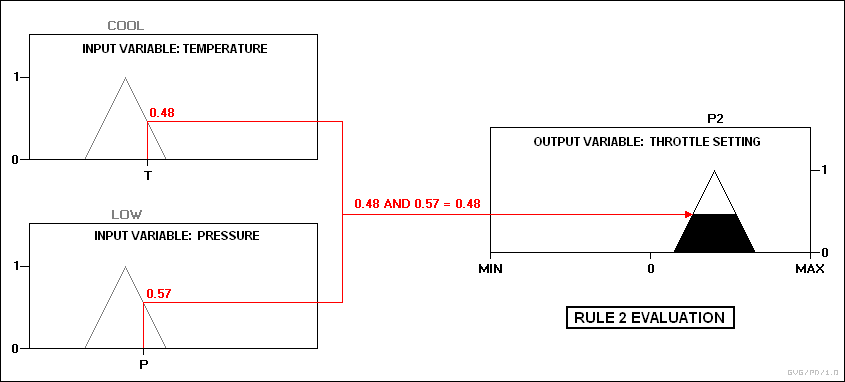Rule 3 is evaluated as follows: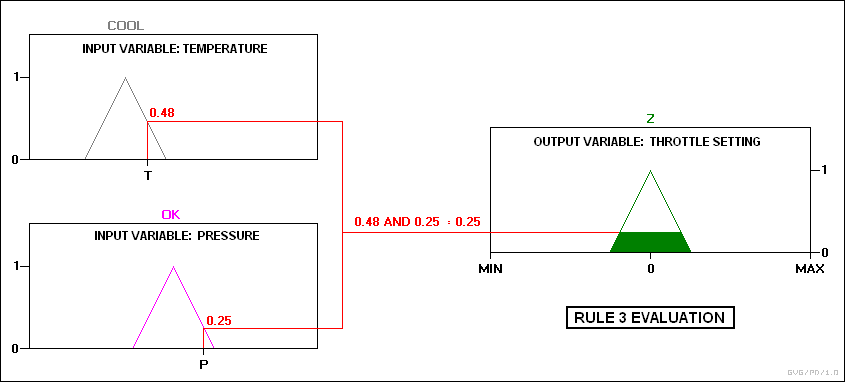The two outputs are then combined: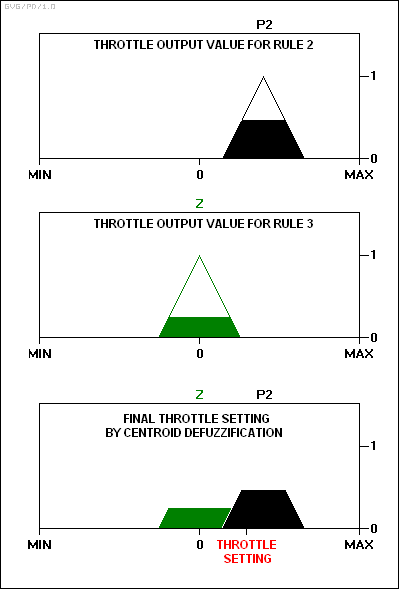The output value will adjust the throttle and then the control cycle will begin again to generate the next value.

##  BUILDING A FUZZY CONTROLLER

* Consider implementing with a microcontroller chip a simple feedback controller:A fuzzy set is defined for the input error variable "e", and the derived change in error from the previous error input, "delta", as well as the "output", as follows:

```   LP:  large positive
SP:  small positive
ZE:  zero
SN:  small negative
LN:  large negative

```
If the error ranges from -1 to +1, with the analog-to-digital converter used having a resolution of 0.25, then the input variable's fuzzy set (which, in this case, also applies to the output variable) can be described very simply as a table, with the error / delta / output values in the top row and the truth values for each membership function arranged in rows beneath:
```   _______________________________________________________________________

-1    -0.75  -0.5   -0.25    0     0.25   0.5    0.75    1
_______________________________________________________________________

mu(LP)      0      0      0      0      0      0     0.3    0.7     1
mu(SP)      0      0      0      0     0.3    0.7     1     0.7    0.3
mu(ZE)      0      0     0.3    0.7     1     0.7    0.3     0      0
mu(SN)     0.3    0.7     1     0.7    0.3     0      0      0      0
mu(LN)      1     0.7    0.3     0      0      0      0      0      0
_______________________________________________________________________

```
This table is a little tricky to interpret. It simply defines each of the elements of the fuzzy set ("LP" through "LN") in terms of the values ("mu") that they have relative to the given values in the top row.

It is important to remember that for the error / delta variables, the top row values are used to generate the mu values, while for the output variable, the mu values are used to generate the top row values.

Suppose this fuzzy system has the following rule base:

```   rule 1:  IF e = ZE AND delta = ZE THEN output = ZE
rule 2:  IF e = ZE AND delta = SP THEN output = SN
rule 3:  IF e = SN AND delta = SN THEN output = LP
rule 4:  IF e = LP OR  delta = LP THEN output = LN

```
These rules are typical for control applications in that the antecedents consist of the logical combination of the error and delta signals, while the consequent is a control command output.

These rules can also be a little tricky to interpret. For example, what rule 1 says is that if the error value is the ZE fuzzy set element and the delta, or change from last error, value is the ZE fuzzy set element, then the output is also the ZE fuzzy set element. What is very tricky is that plugging actual numeric values into this rule does not give, at least directly, an actual value for the output setting.

The value for the output setting is defined by rule 1 as "ZE". Looking at the fuzzy value table shows that "ZE" has its maximum value corresponding to the value of "0" on the top row of the table, and so this rule always gives an output setting of 0. Similarly, rule 2 always gives an output setting of "SN" or -0.5, rule 3 always gives an output setting of "LP" or 1, and rule 4 always gives an output setting of "LN" or -1.

The thing to remember is that the actual output is a combination of the outputs of these four rules, weighted by the values by the appropriate mu values provided by the rule calculation, using the centroid calculation:

```   SUM( I = 1 TO 4 OF ( mu(I) * output(I) ) ) / SUM( I = 1 TO 4 OF mu(I) )

```
The result of this calculation gives the actual output setting.

* Now, suppose that at a given time we have:

```   error = 0.25
delta = 0.5
```
Then the corresponding mu values can be obtained by simply taking them from the appropriate columns of the fuzzy set table:
```   _________________________________

error = 0.25   delta = 0.5
_________________________________

mu(LP)       0            0.3
mu(SP)      0.7            1
mu(ZE)      0.7           0.3
mu(SN)       0             0
mu(LN)       0             0
_________________________________

```
These values can then be plugged into the rules to give output values. Taking the first rule:
```   rule 1:  IF e = ZE AND delta = ZE THEN output = ZE

```
The weighting for the output, "mu(1)", is produced with the simple calculation:
```   mu(1) = MIN( 0.7, 0.3 ) = 0.3

```
As mentioned above, the output value of this rule is always 1:
```   output(1) = 0

```
The other rules give:
```   rule 2:  IF e = ZE AND delta = SP THEN output = SN

mu(2)     = MIN( 0.7, 1 ) = 0.7
output(2) = -0.5

rule 3: IF e = SN AND delta = SN THEN output = LP

mu(3)     = MIN( 0.0, 0.0 ) = 0
output(3) = 1

rule 4: IF e = LP OR  delta = LP THEN output = LN

mu(4)     = MAX( 0.0, 0.3 ) = 0.3
output(4) = -1

```
The centroid computation yields:
```     mu(1)*output(1) + mu(2)*output(2) + mu(3)*output(3) + mu(3)*output(3)
_____________________________________________________________________

mu(1) + mu(2) + mu(3) + mu(4)

=   ( 0.3 * 0 ) + ( 0.7 * -0.5 ) + ( 0 * 1 ) + ( 0.3 * -1 )
_______________________________________________________

0.3 + 0.7 + 0 + 0.3

0 - 0.35 + 0 - 0.3
=   __________________

1.3

= -0.65 / 1.3

= -0.5

```
-- for the final control output. . Of course the hard part is figuring out what rules actually work correctly in practice.

* If you have problems figuring out the centroid equation, remember that a centroid is defined by summing all the moments (location times mass) around the center of gravity and equating the sum to zero. So if X0 is the center of gravity, Xi is the location of each mass, and Mi is each mass, this gives:

```   0 = ( X1 - X0 ) * M1 + ( X2 - X0 ) * M2 + ... + ( Xn - X0 ) * Mn

0 = ( X1 * M1 + X2 * M2 + ... + Xn * Mn ) - X0 * ( M1 + M2 + ... + Mn )

X0 * ( M1 + M2 + ... + Mn ) = ( X1 * M1 + X2 * M2 + ... + Xn * Mn )

( X1 * M1 + X2 * M2 + ... + Xn * Mn )
X0 =    _____________________________________

( M1 + M2 + ... + Mn )

```
In our example, the values of mu correspond to the masses, and the values of X to location of the masses.

##  HISTORY & APPLICATIONS

* Fuzzy logic was first proposed by Lotfi A. Zadeh of the University of California at Berkeley in a 1965 paper. He elaborated on his ideas in a 1973 paper that introduced the concept of "linguistic variables", which in this article equates to a variable defined as a fuzzy set. Other research followed, with the first industrial application, a cement kiln built in Denmark, coming on line in 1975.

Fuzzy systems were largely ignored in the US because they were associated with artificial intelligence, a field that periodically oversells itself and which did so in a big way in the mid-1980s, resulting in a lack of credibility in the commercial domain.

The Japanese did not have this prejudice. Interest in fuzzy systems was sparked by Seiji Yasunobu and Soji Miyamoto of Hitachi, who in 1985 provided simulations that demonstrated the superiority of fuzzy control systems for the Sendai railway. Their ideas were adopted, and fuzzy systems were used to control accelerating, braking, and stopping when the line opened in 1987.

Another event in 1987 helped promote interest in fuzzy systems. During a international meeting of fuzzy researchers in Tokyo that year, Takeshi Yamakawa demonstrated the use of fuzzy control, through a set of simple dedicated fuzzy logic chips, in an "inverted pendulum" experiment. This is a classic control problem, in which a vehicle tries to keep a pole mounted on its top by a hinge upright by moving back and forth.

Observers were impressed with this demonstration, as well as later experiments by Yamakawa in which he mounted a wine glass containing water or even a live mouse to the top of the pendulum. The system maintained stability in both cases. Yamakawa eventually went on to organize his own fuzzy-systems research lab to help exploit his patents in the field.

Following such demonstrations, the Japanese became infatuated with fuzzy systems, developing them for both industrial and consumer applications. In 1988 they established the Laboratory for International Fuzzy Engineering (LIFE), a cooperative arrangement between 48 companies to pursue fuzzy research. Japanese companies developed a wide range of products using fuzzy logic, ranging from washing machines to autofocus cameras and industrial air conditioners.

Some work was also performed on fuzzy logic systems in the US and Europe, and a number of products were developed using fuzzy logic controllers. However, little has been said about the technology in recent years, which implies that it has either become such an ordinary tool that it is no longer worth much comment, or it turned out to be an industrial fad that has now generally died out.

##  COMMENTS, SOURCES, & REVISION HISTORY

* I have no background in control systems nor in fuzzy logic, but something about fuzzy logic intrigues me. I had accumulated notes on the subject off and on for a few years, and then reached a threshold where I decided to merge them into my own tutorial, focusing on control applications.

• "Designing With Fuzzy Logic" by Kevin Self, IEEE SPECTRUM, November 1990, 42:44,105.

• "Fuzzy Fundamentals" by Earl Cox, IEEE SPECTRUM, October 1992, 58:61. Notes from this article constitute the core of this tutorial.

• "Fuzzy Logic Flowers In Japan" by Daniel G. Schwartz & George J. Klir, IEEE SPECTRUM, July 1992, 32:35.

• "Clear Thinking On Fuzzy Logic" by Lawrence A. Berardinis, MACHINE DESIGN, 23 April 1992, 46:52.

• "Fuzzy Controller Challenges 8-bit MCUs", COMPUTER DESIGN, November 1995.

• "Dishwasher Cleans Up With Fuzzy Logic", MACHINE DESIGN, 23 March 1995.

• "How To Design Fuzzy Logic Controllers", MACHINE DESIGN, 26 November 1992.

• "A Case For Fuzzy Logic" by Byron Miller, EMBEDDED SYSTEMS PROGRAMMING, December 1995, 42:70.

* Revision history:

```   v1.0   / 23 dec 95 / gvg
v1.1   / 06 dec 98 / gvg / Minor cosmetic update.
v1.2   / 01 oct 00 / gvg / Minor cosmetic update.
v1.0.3 / 01 jul 02 / gvg / Cosmetic update and simplification.
v1.0.4 / 01 jun 03 / gvg / Minor update with clarifications.
```
BACK_TO_TOP